# Introduction¶

We will create the email spam filter model using deep learning and evaluate the model with other currently popular machine learning methods like xgboost, random forest, svm etc.

For this sample project, we will use Enron dataset in English. However this approach works well for other languages also which i had empiricially tested in my job.

This approach combines unsupervised learning with Supervised learning. We will generate the features in unsupervised way using TF-IDF algorithm and then use this to features to train Models on labeled enron data.

The code and data for this project can be obtained at : https://github.com/sanjaymeena/Deep-Learning-based-Spam-Filter

The broad steps can be divided as :

## 1. Preprocessing:¶

Here we will generate a pandas dataframe from the enron dataset . We will tokenize and also do some data analysis

## 2. Features Generation (Unsupervised Learning)¶

We will use TF-IDF as features to be used for training the models.

## 3. Model Training¶

• We will train a 3-layered deep learning model.
• We will also train Random forest, SVM and Xgboost for comparison purpose.
• We will the same tf-idf features for all the models

## 4. Result Analysis and iterate to improve the performnce¶

• We will present our results in nice and informative way to provide good comparison information.

# 1. Preprocessing¶

In [ ]:


In :
# Load required libraries
import matplotlib.pyplot as plt
import numpy as np
import pandas as pd
import os
import time
import pickle
import seaborn as sns
import sys
sys.setrecursionlimit(1500)
%matplotlib inline


# Preparing Enron Data¶

We will extract and load the Enron spam data in Pandas Dataframe.

Enron data combined with Spam assasin dataset has been obtained from : https://www.cs.bgu.ac.il/~elhadad/nlp16/spam_classifier.html and I also used their code to process the data into Pandas dataframe

In :
def progress(i, end_val, bar_length=50):
'''
Print a progress bar of the form: Percent: [#####      ]
i is the current progress value expected in a range [0..end_val]
bar_length is the width of the progress bar on the screen.
'''
percent = float(i) / end_val
hashes = '#' * int(round(percent * bar_length))
spaces = ' ' * (bar_length - len(hashes))
sys.stdout.write("\rPercent: [{0}] {1}%".format(hashes + spaces, int(round(percent * 100))))
sys.stdout.flush()

NEWLINE = '\n'

In :
HAM = 'ham'
SPAM = 'spam'

SOURCES = [
('../data/enron//spam',        SPAM),
('../data/enron//easy_ham',    HAM),
('../data/enron//hard_ham',    HAM),
('../data/enron//beck-s',      HAM),
('../data/enron//farmer-d',    HAM),
('../data/enron//kaminski-v',  HAM),
('../data/enron//kitchen-l',   HAM),
('../data/enron//lokay-m',     HAM),
('../data/enron//williams-w3', HAM),
('../data/enron//BG',          SPAM),
('../data/enron//GP',          SPAM),
('../data/enron//SH',          SPAM)
]

SKIP_FILES = {'cmds'}
NEWLINE="\n"

'''
Generator of pairs (filename, filecontent)
for all files below path whose name is not in SKIP_FILES.
The content of the file is of the form:
<emptyline>
body...
This skips the headers and returns body only.
'''
for root, dir_names, file_names in os.walk(path):
for path in dir_names:
for file_name in file_names:
if file_name not in SKIP_FILES:
file_path = os.path.join(root, file_name)
if os.path.isfile(file_path):
f = open(file_path, encoding="latin-1")
for line in f:
lines.append(line)
elif line == NEWLINE:
f.close()
content = NEWLINE.join(lines)
yield file_path, content

def build_data_frame(l, path, classification):
rows = []
index = []
for i, (file_name, text) in enumerate(read_files(path)):
if ((i+l) % 100 == 0):
progress(i+l, 58910, 50)
rows.append({'text': text, 'label': classification,'file':file_name})
index.append(file_name)

data_frame = pd.DataFrame(rows, index=index)
return data_frame, len(rows)

data = pd.DataFrame({'text': [], 'label': [],'file':[]})
l = 0
for path, classification in SOURCES:
data_frame, nrows = build_data_frame(l, path, classification)
data = data.append(data_frame)
l += nrows
data = data.reindex(np.random.permutation(data.index))
return data

In :
# We will load the Email spam dataset into Panadas dataframe here .

Percent: [################################################  ] 96%
In :
# We change the dataframe index from filenames to indices here.

In :
new_index=[x for x in range(len(data))]
data.index=new_index


We will add two more columns to our dataframe for tokenized text and token count.

In :
def token_count(row):
'returns token count'
text=row['tokenized_text']
length=len(text.split())
return length

def tokenize(row):
"tokenize the text using default space tokenizer"
text=row['text']
lines=(line for line in text.split(NEWLINE) )
tokenized=""
for sentence in lines:
tokenized+= " ".join(tok for tok in sentence.split())


We will use apply functions on dataframe to add the columns for :

* Tokenized text
* Token Count
* Language


Language column in this case is not necessary as we only have english text. However this approach is good for properly dealing with multi lingual data.

In :
data['tokenized_text']=data.apply(tokenize, axis=1)

In :
data['token_count']=data.apply(token_count, axis=1)

In :
data['lang']='en'


Let's look at how our dataframe looks like

In :
data.head()

Out:
file label text tokenized_text token_count lang
0 ../data/enron//kaminski-v/personal/340 ham Dear Vince-\n\n\n\nI am soooo gland to see you... Dear Vince-I am soooo gland to see you get the... 38 en
1 ../data/enron//williams-w3/hr/23 ham I guess I forgot to send this to you. Cynthia ... I guess I forgot to send this to you. Cynthia ... 180 en
2 ../data/enron//BG/2004/09/1095440603.31976_48.txt spam \n\nFROM THE DESK OF LUKE DOMA \n\nWEMA BANK P... FROM THE DESK OF LUKE DOMAWEMA BANK PLC,LAGOS-... 350 en
3 ../data/enron//GP/part7/msg10809.eml spam <!DOCTYPE HTML PUBLIC "-//W3C//DTD HTML 4.0 Tr... <!DOCTYPE HTML PUBLIC "-//W3C//DTD HTML 4.0 Tr... 15 en
4 ../data/enron//SH/HP/prodmsg.2.437295.200572 spam This is a multi-part message in MIME format.\n... This is a multi-part message in MIME format.--... 328 en
In :
# Lets look at some information related to the data

In :
df=data
print("total emails : ", len(df))
print  ("total spam emails : ", len(df[df['label']=='spam']) )
print  ("total normal emails : ", len(df[df['label']=='ham']) )

total emails :  56513
total spam emails :  32974
total normal emails :  23539

In [ ]:



### Plot of Emails with Langage and Email type¶

In :
df1 = df.groupby(['lang','label'])['label','lang'].size().unstack()

ax=df1.plot(kind='bar')
ax.set_ylabel("Total Emails")
ax.set_xlabel("Language")
ax.set_title("Plot of Emails count with languages and email type")

Out:
<matplotlib.text.Text at 0x11279f518>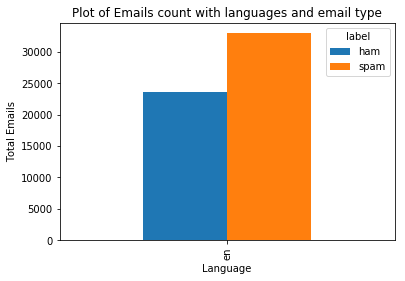In :
bins = [0,100,200,300,350,400,500,600,800,1000,1500,2000,3000,4000,5000,6000,10000,20000]

fig, axes = plt.subplots(nrows=1, ncols=2,figsize=(12, 6))

df_sub=df[ (df['lang']=='en') & (df['label']=='ham')]
df1 = df_sub.groupby(pd.cut(df_sub['token_count'], bins=bins)).token_count.count()
df1.index=[a.right for a in df1.index]
res1=df1.plot(kind='bar',ax=axes)
res1.set_xlabel('Email tokens length')
res1.set_ylabel('Frequency')
res1.set_title('Token length Vs Frequency for Enron Normal Emails')

df_sub=df[ (df['lang']=='en') & (df['label']=='spam')]
df1 = df_sub.groupby(pd.cut(df_sub['token_count'], bins=bins)).token_count.count()
df1.index=[a.right for a in df1.index]
res2=df1.plot(kind='bar',ax=axes)
res2.set_xlabel('Email tokens length')
res2.set_ylabel('Frequency')
res2.set_title('Token length Vs Frequency for Enron Spam Emails')

Out:
<matplotlib.text.Text at 0x105ef6550>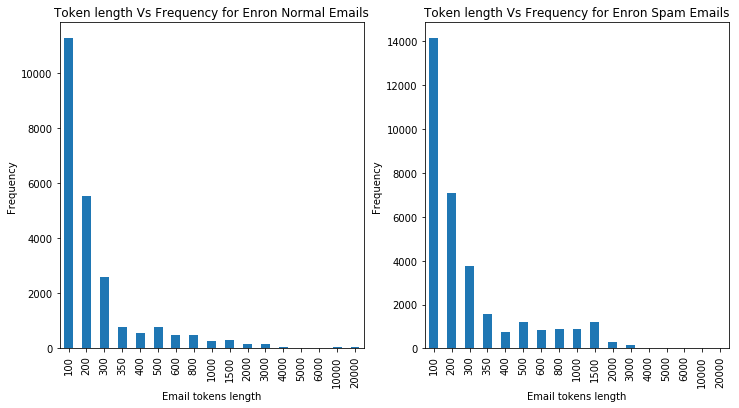# Prepare training and test data¶

We will split data into test data and data for model training and validation. We do this step to keep test data out of both tf-idf and classifier models.

We will keep 10000 emails for testing and rest for the model building process.

We shuffle the data in the dataframe first.

In :
# We randomize the rows to subset the dataframe
df.reset_index(inplace=True)
df=df.reindex(np.random.permutation(df.index))

In :
len_unseen=10000
df_unseen_test= df.iloc[:len_unseen]
df_model = df.iloc[len_unseen:]

print('total emails for unseen test data : ', len(df_unseen_test))
print('\t total spam emails for enron  : ', len(df_unseen_test[(df_unseen_test['lang']=='en') & (df_unseen_test['label']=='spam')]))
print('\t total normal emails for enron  : ', len(df_unseen_test[(df_unseen_test['lang']=='en') & (df_unseen_test['label']=='ham')]))
print()

print('total emails for model training/validation : ', len(df_model))
print('\t total spam emails for enron  : ', len(df_model[(df_model['lang']=='en') & (df_model['label']=='spam')]))
print('\t total normal emails for enron  : ', len(df_model[(df_model['lang']=='en') & (df_model['label']=='ham')]))

total emails for unseen test data :  10000
total spam emails for enron  :  5823
total normal emails for enron  :  4177

total emails for model training/validation :  46513
total spam emails for enron  :  27151
total normal emails for enron  :  19362


# Train Machine Learning Models¶

In :
## Deep Learning Model


We will build our deep learning model using Keras library with tensorflow as backend.

In :
import keras

from keras.layers import Input, Dense
from keras.layers import Input, Dense
from keras.models import Model
from keras import regularizers

from keras.preprocessing.text import Tokenizer
from keras.models import Sequential
from keras.layers import Dense, Dropout, Activation
from keras.callbacks import ModelCheckpoint, TensorBoard

Using TensorFlow backend.

In :
import sklearn
from sklearn import metrics
from sklearn import svm
from sklearn.externals import joblib
from sklearn.preprocessing import LabelEncoder

In [ ]:



# Create tf-idf model from the data¶

We will create tf-idf model with keras

In :
# max number of features
num_max = 4000

In :
def train_tf_idf_model(texts):
"train tf idf model "
tic = time.process_time()

tok = Tokenizer(num_words=num_max)
tok.fit_on_texts(texts)
toc = time.process_time()

print (" -----total Computation time = " + str((toc - tic)) + " seconds")

def prepare_model_input(tfidf_model,dataframe,mode='tfidf'):

"function to prepare data input features using tfidf model"
tic = time.process_time()

le = LabelEncoder()
sample_texts = list(dataframe['tokenized_text'])
sample_texts = [' '.join(x.split()) for x in sample_texts]

targets=list(dataframe['label'])
targets = [1. if x=='spam' else 0. for x in targets]
sample_target = le.fit_transform(targets)

if mode=='tfidf':
sample_texts=tfidf_model.texts_to_matrix(sample_texts,mode='tfidf')
else:
sample_texts=tfidf_model.texts_to_matrix(sample_texts)

toc = time.process_time()

print('shape of labels: ', sample_target.shape)
print('shape of data: ', sample_texts.shape)

print (" -----total Computation time for preparing model data = " + str((toc - tic)) + " seconds")

return sample_texts,sample_target

In [ ]:


In :
texts=list(df_model['tokenized_text'])
tfidf_model=train_tf_idf_model(texts)

 -----total Computation time = 19.723424 seconds

In :
# prepare model input data
mat_texts,tags=prepare_model_input(tfidf_model,df_model,mode='tfidf')

shape of labels:  (46513,)
shape of data:  (46513, 4000)
-----total Computation time for preparing model data = 34.13841300000001 seconds

In [ ]:



# Split Train/validation data¶

We will use 85% for training, 15% for validation.

In :
from sklearn.model_selection import train_test_split

X_train, X_val, y_train, y_val = train_test_split(mat_texts, tags, test_size=0.15)
print ('train data shape: ', X_train.shape, y_train.shape)
print ('validation data shape :' , X_val.shape, y_val.shape)

train data shape:  (39536, 4000) (39536,)
validation data shape : (6977, 4000) (6977,)


# Build models¶

## Deep learning model¶

We will build our 3 layer deep learning model using Keras and tensorflow.

### Network¶

Input -> L1 : (Linear -> Relu) -> L2: (Linear -> Relu)-> (Linear -> Sigmoid)

• Layer L1 has 512 neurons with Relu activation
• Layer L2 has 256 neurons with Relu activation

• Regularization : We use dropout with probability 0.5 for L1, L2 to prevent overfitting

• Loss Function : binary cross entropy
• Optimizer : We use Adam optimizer for gradient descent estimation (faster optimization)
• Data Shuffling : Data shuffling is set to true
• Batch Size : 64
• Learning Rate = 0.001
In :
## Define and initialize the network

model_save_path="checkpoints/spam_detector_enron_model.h5"

In :
def get_simple_model():
model = Sequential()
model.summary()
model.compile(loss='binary_crossentropy',
metrics=['acc',keras.metrics.binary_accuracy])
print('compile done')
return model

def check_model(model,x,y,epochs=2):
history=model.fit(x,y,batch_size=32,epochs=epochs,verbose=1,shuffle=True,validation_split=0.2,
callbacks=[checkpointer, tensorboard]).history
return history

def check_model2(model,x_train,y_train,x_val,y_val,epochs=10):
history=model.fit(x_train,y_train,batch_size=64,
epochs=epochs,verbose=1,
shuffle=True,
validation_data=(x_val, y_val),
callbacks=[checkpointer, tensorboard]).history
return history

# define checkpointer
checkpointer = ModelCheckpoint(filepath=model_save_path,
verbose=1,
save_best_only=True)

# define tensorboard
tensorboard = TensorBoard(log_dir='./logs',
histogram_freq=0,
write_graph=True,
write_images=True)

# define the predict function for the deep learning model for later use
def predict(data):
result=spam_model_dl.predict(data)
prediction = [round(x) for x in result]
return prediction

In :
## Train the model

In :
# get the compiled model
model = get_simple_model()

# history=check_model(m,mat_texts,tags,epochs=10)
history=check_model2(model,X_train,y_train,X_val,y_val,epochs=10)

_________________________________________________________________
Layer (type)                 Output Shape              Param #
=================================================================
dense_1 (Dense)              (None, 512)               2048512
_________________________________________________________________
dropout_1 (Dropout)          (None, 512)               0
_________________________________________________________________
dense_2 (Dense)              (None, 256)               131328
_________________________________________________________________
dropout_2 (Dropout)          (None, 256)               0
_________________________________________________________________
dense_3 (Dense)              (None, 1)                 257
=================================================================
Total params: 2,180,097
Trainable params: 2,180,097
Non-trainable params: 0
_________________________________________________________________
compile done
Train on 39536 samples, validate on 6977 samples
Epoch 1/10
39424/39536 [============================>.] - ETA: 0s - loss: 0.0825 - acc: 0.9791 - binary_accuracy: 0.9791Epoch 00000: val_loss improved from inf to 0.04133, saving model to checkpoints/spam_detector_enron_model.h5
39536/39536 [==============================] - 27s - loss: 0.0823 - acc: 0.9791 - binary_accuracy: 0.9791 - val_loss: 0.0413 - val_acc: 0.9911 - val_binary_accuracy: 0.9911
Epoch 2/10
39424/39536 [============================>.] - ETA: 0s - loss: 0.0394 - acc: 0.9925 - binary_accuracy: 0.9925Epoch 00001: val_loss improved from 0.04133 to 0.03843, saving model to checkpoints/spam_detector_enron_model.h5
39536/39536 [==============================] - 26s - loss: 0.0393 - acc: 0.9925 - binary_accuracy: 0.9925 - val_loss: 0.0384 - val_acc: 0.9931 - val_binary_accuracy: 0.9931
Epoch 3/10
39424/39536 [============================>.] - ETA: 0s - loss: 0.0198 - acc: 0.9959 - binary_accuracy: 0.9959Epoch 00002: val_loss did not improve
39536/39536 [==============================] - 27s - loss: 0.0198 - acc: 0.9959 - binary_accuracy: 0.9959 - val_loss: 0.0490 - val_acc: 0.9897 - val_binary_accuracy: 0.9897
Epoch 4/10
39424/39536 [============================>.] - ETA: 0s - loss: 0.0171 - acc: 0.9968 - binary_accuracy: 0.9968- ETA: 2s - loss: 0.0182 - acc: 0.Epoch 00003: val_loss did not improve
39536/39536 [==============================] - 28s - loss: 0.0170 - acc: 0.9968 - binary_accuracy: 0.9968 - val_loss: 0.0454 - val_acc: 0.9905 - val_binary_accuracy: 0.9905
Epoch 5/10
39488/39536 [============================>.] - ETA: 0s - loss: 0.0185 - acc: 0.9965 - binary_accuracy: 0.9965Epoch 00004: val_loss did not improve
39536/39536 [==============================] - 27s - loss: 0.0186 - acc: 0.9965 - binary_accuracy: 0.9965 - val_loss: 0.0472 - val_acc: 0.9921 - val_binary_accuracy: 0.9921
Epoch 6/10
39488/39536 [============================>.] - ETA: 0s - loss: 0.0147 - acc: 0.9974 - binary_accuracy: 0.9974Epoch 00005: val_loss did not improve
39536/39536 [==============================] - 28s - loss: 0.0147 - acc: 0.9974 - binary_accuracy: 0.9974 - val_loss: 0.0403 - val_acc: 0.9936 - val_binary_accuracy: 0.9936
Epoch 7/10
39488/39536 [============================>.] - ETA: 0s - loss: 0.0129 - acc: 0.9980 - binary_accuracy: 0.9980Epoch 00006: val_loss did not improve
39536/39536 [==============================] - 27s - loss: 0.0129 - acc: 0.9980 - binary_accuracy: 0.9980 - val_loss: 0.0490 - val_acc: 0.9908 - val_binary_accuracy: 0.9908
Epoch 8/10
39488/39536 [============================>.] - ETA: 0s - loss: 0.0107 - acc: 0.9985 - binary_accuracy: 0.9985Epoch 00007: val_loss improved from 0.03843 to 0.03611, saving model to checkpoints/spam_detector_enron_model.h5
39536/39536 [==============================] - 26s - loss: 0.0107 - acc: 0.9985 - binary_accuracy: 0.9985 - val_loss: 0.0361 - val_acc: 0.9937 - val_binary_accuracy: 0.9937
Epoch 9/10
39424/39536 [============================>.] - ETA: 0s - loss: 0.0127 - acc: 0.9982 - binary_accuracy: 0.9982Epoch 00008: val_loss did not improve
39536/39536 [==============================] - 26s - loss: 0.0127 - acc: 0.9982 - binary_accuracy: 0.9982 - val_loss: 0.0606 - val_acc: 0.9920 - val_binary_accuracy: 0.9920
Epoch 10/10
39424/39536 [============================>.] - ETA: 0s - loss: 0.0103 - acc: 0.9988 - binary_accuracy: 0.9988Epoch 00009: val_loss did not improve
39536/39536 [==============================] - 27s - loss: 0.0103 - acc: 0.9988 - binary_accuracy: 0.9988 - val_loss: 0.0561 - val_acc: 0.9925 - val_binary_accuracy: 0.9925


The results on validation data looks very good. Lets plot the loss on train and validation data

In :
plt.plot(history['loss'])
plt.plot(history['val_loss'])
plt.title('Email Spam Filter Model loss')
plt.ylabel('loss')
plt.xlabel('epoch')
plt.legend(['train', 'test'], loc='upper right');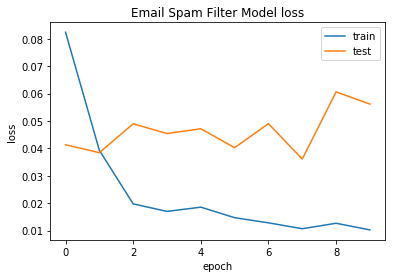## Other Machine Learning Models¶

We will build 3 more models and compare the performance in the same way. For this purpose we will use the same tf-idf as input feature . We will train following models :

• SVM
• Random Forest
• XGboost
In :



### Lets train the svm model¶

In :
spam_model_svm = svm.SVC(verbose=1)
spam_model_svm.fit(X_train,y_train)

[LibSVM]
Out:
SVC(C=1.0, cache_size=200, class_weight=None, coef0=0.0,
decision_function_shape='ovr', degree=3, gamma='auto', kernel='rbf',
max_iter=-1, probability=False, random_state=None, shrinking=True,
tol=0.001, verbose=1)

### Lets build random forest¶

In :
from sklearn.ensemble import RandomForestClassifier

In :
spam_model_rf = RandomForestClassifier(n_jobs=2, random_state=0,n_estimators=50)

# Train the Classifier to take the training features and learn how they relate
# to the training y (the species)
spam_model_rf.fit(X_train,y_train)

Out:
RandomForestClassifier(bootstrap=True, class_weight=None, criterion='gini',
max_depth=None, max_features='auto', max_leaf_nodes=None,
min_impurity_decrease=0.0, min_impurity_split=None,
min_samples_leaf=1, min_samples_split=2,
min_weight_fraction_leaf=0.0, n_estimators=50, n_jobs=2,
oob_score=False, random_state=0, verbose=0, warm_start=False)

### Lets train xgboost model¶

In :
# Build xgboost also
import xgboost as xgb

In :
spam_model_xgboost = xgb.XGBClassifier()
spam_model_xgboost.fit(X_train,y_train)

Out:
XGBClassifier(base_score=0.5, booster='gbtree', colsample_bylevel=1,
colsample_bytree=1, gamma=0, learning_rate=0.1, max_delta_step=0,
max_depth=3, min_child_weight=1, missing=None, n_estimators=100,
reg_alpha=0, reg_lambda=1, scale_pos_weight=1, seed=None,
silent=True, subsample=1)
In [ ]:



# Evaluate Model Performance¶

## Lets prepare test data¶

In [ ]:
sample_texts,sample_target=prepare_model_input(tfidf_model,df_unseen_test,mode='')

In :
# lets write a function to create the dataframe of the results from all the models

In :
model_dict={}
model_dict['random_forest']=spam_model_rf
model_dict['svm']=spam_model_svm
model_dict['deep_learning']=spam_model_dl
model_dict['xgboost']=spam_model_xgboost

def getResults(model_dict,sample_texts,sample_target):
'''
Get results from different models
'''
results=[]

results_cm={}

for name,model in model_dict.items():
#         print(name)
tic1 = time.process_time()
if name in 'deep_learning':
predicted_sample = predict(sample_texts)
else:
predicted_sample = model.predict(sample_texts)
toc1 = time.process_time()
#         print(predicted_sample)

cm=sklearn.metrics.confusion_matrix(sample_target, predicted_sample)
results_cm[name]=cm

total=len(predicted_sample)
TP = cm
FP = cm
FN = cm
TN = cm

time_taken=round(toc1 - tic1,4)
res=sklearn.metrics.precision_recall_fscore_support(sample_target, predicted_sample)
results.append([name,np.mean(res),np.mean(res),np.mean(res),total,TP,FP,FN,TN,str(time_taken)] )

df_cols=['model','precision','recall','f1_score','Total_samples','TP','FP','FN','TN','execution_time']
result_df=pd.DataFrame(results,columns=df_cols)

return result_df,results_cm



## Results¶

In :
result_df,results_cm= getResults(model_dict,sample_texts,sample_target)
result_df

Out:
model precision recall f1_score Total_samples TP FP FN TN execution_time
0 random_forest 0.887624 0.896754 0.890721 10000 3743 338 732 5187 0.5935
1 svm 0.935807 0.947646 0.939390 10000 4026 55 540 5379 318.8586
2 deep_learning 0.990649 0.990723 0.990686 10000 4037 44 46 5873 5.4259
3 xgboost 0.882452 0.875857 0.878744 10000 3398 683 479 5440 0.4664

As we see, deep learning model does very well on the test data. The results from other models are close. I have tried this approach over multiple language emails and deep learning model is very consistent with the performance. XGboost also does very well. Please note that i have not optimized random forest and SVM much beyond the defaults. So they may have better performance with tuning.

### Plot confusion Matrix for all the models¶

In :
def plot_heatmap(cm,title):
df_cm2 = pd.DataFrame(cm, index = ['normal', 'spam'])
df_cm2.columns=['normal','spam']

ax = plt.axes()
sns.heatmap(df_cm2, annot=True, fmt="d", linewidths=.5,ax=ax)
ax.set_title(title)
plt.show()

return



### CM for Deep Learning Model¶

In :
plot_heatmap(results_cm['deep_learning'],'Deep Learning')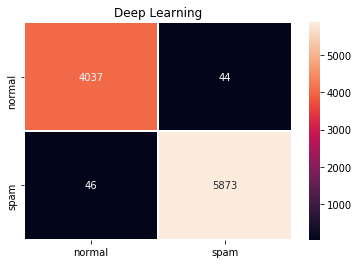### CM for SVM Model¶

In :
plot_heatmap(results_cm['svm'],'SVM')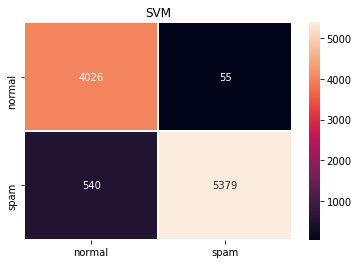### CM for Random Forest Model¶

In :
plot_heatmap(results_cm['random_forest'],'Random Forest')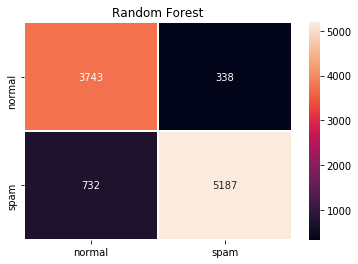### CM for Xgboost¶

In :
plot_heatmap(results_cm['xgboost'],'xgboost')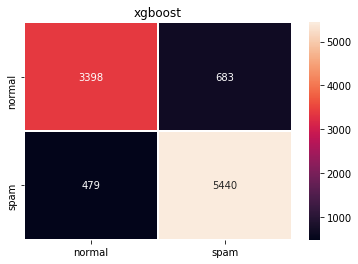In [ ]:



The code and data for this project can be obtained at : https://github.com/sanjaymeena/Deep-Learning-based-Spam-Filter

In [ ]: